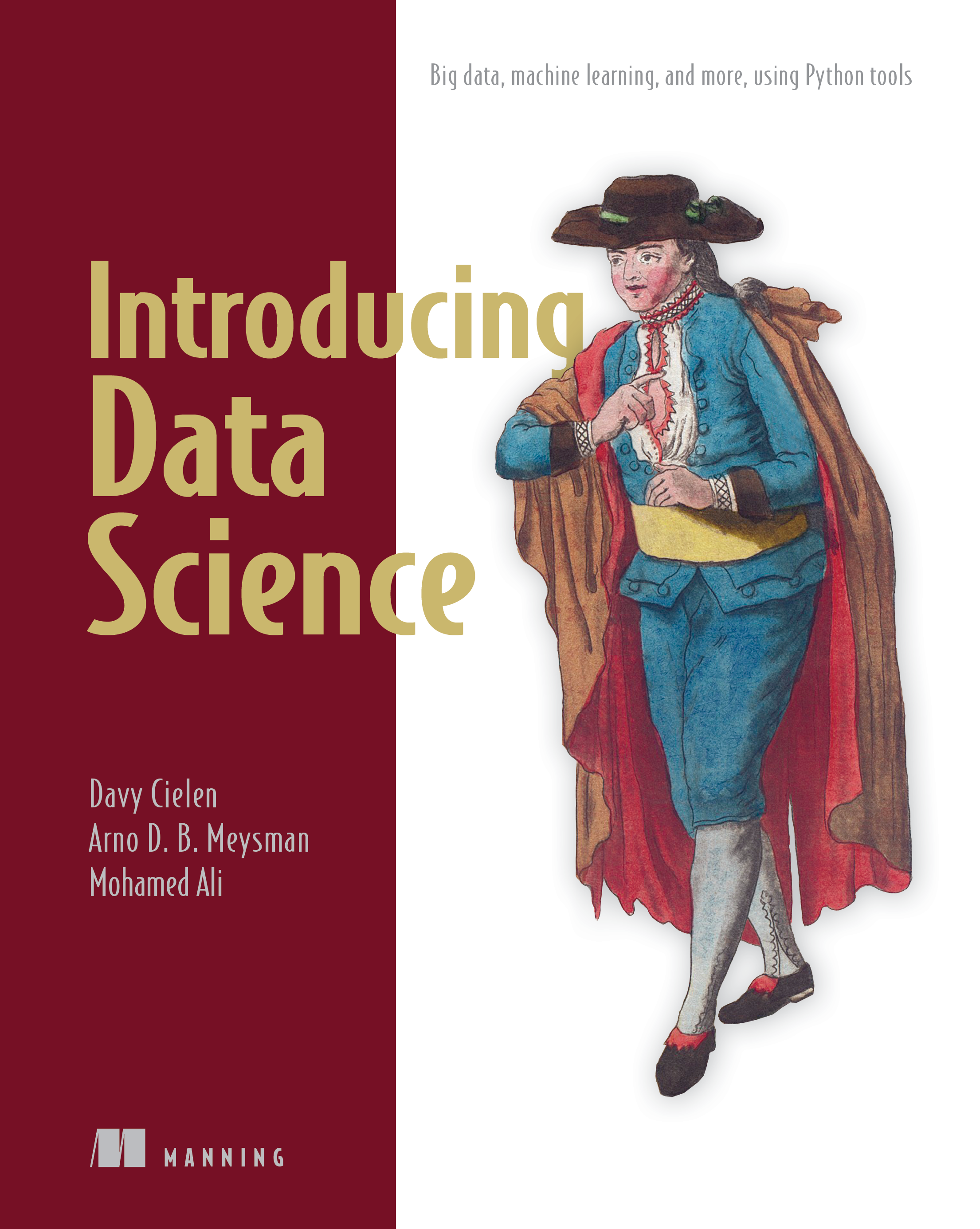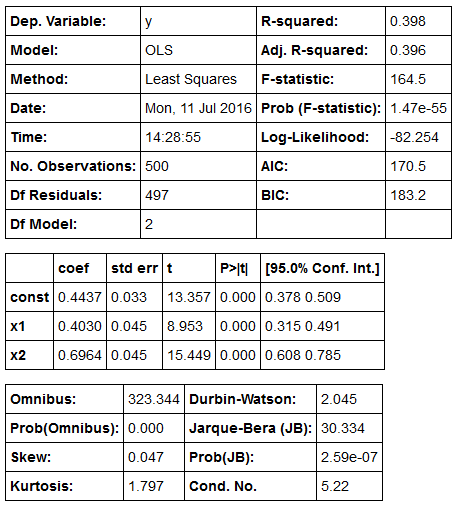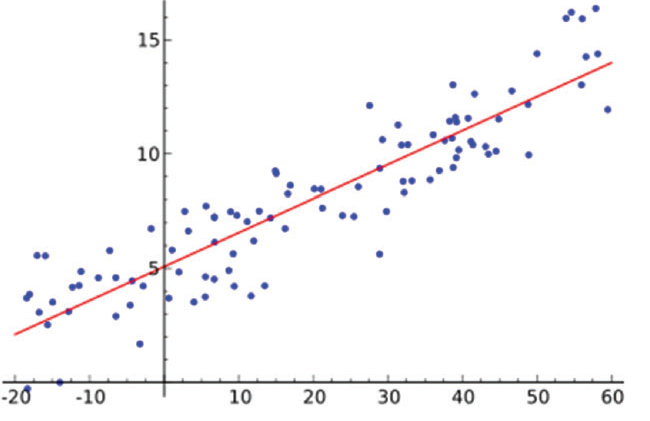Introducing Data Science ErrataThank you for purchasing Introducing Data Science. Please post any errors, other than those listed below, in the book's Author Online Forum. We'll compile a comprehensive list and publish it here for everyone's convenience. Thank you!

Last updated: August 22, 2016

• ##### Page 49, Listing 2.1:

While not a true error, it has been pointed out the model would make more sense if we add a constant to the formula. For demonstration purposes we left this out but if you like, you can do so with minimum effort. The following code:

import statsmodels.api as sm

import numpy as np

predictors = np.random.random(1000).reshape(500,2)

target = predictors.dot(np.array([0.4, 0.6])) + np.random.random(500)

lmRegModel = sm.OLS(target,predictors)

result = lmRegModel.fit()

result.summary()

Now becomes:

import statsmodels.api as sm

import numpy as np

predictors = np.random.random(1000).reshape(500,2)

target = predictors.dot(np.array([0.4, 0.6])) + np.random.random(500)

lmRegModel = sm.OLS(target,predictors)

result = lmRegModel.fit()

result.summary()

A single line of code is sufficient to add this constant. Our summary now shows coefficients way closer to the true imputed values.• ##### Page 50, Figure 2.22:

The following is not an error but a warning to avoid confusion. The regression chart shown (figure 2.22) is unrelated to the fictional code example before it. This chart merely shows what a regresion line could look like when working with only two variables (simple regression). The previous code example is multiple regression and would have a regression line in a 3-dimensional space. Obviously this would have been harder to interpret when first exposed to the concept of a regression line so we opted to show a simple regression instead.